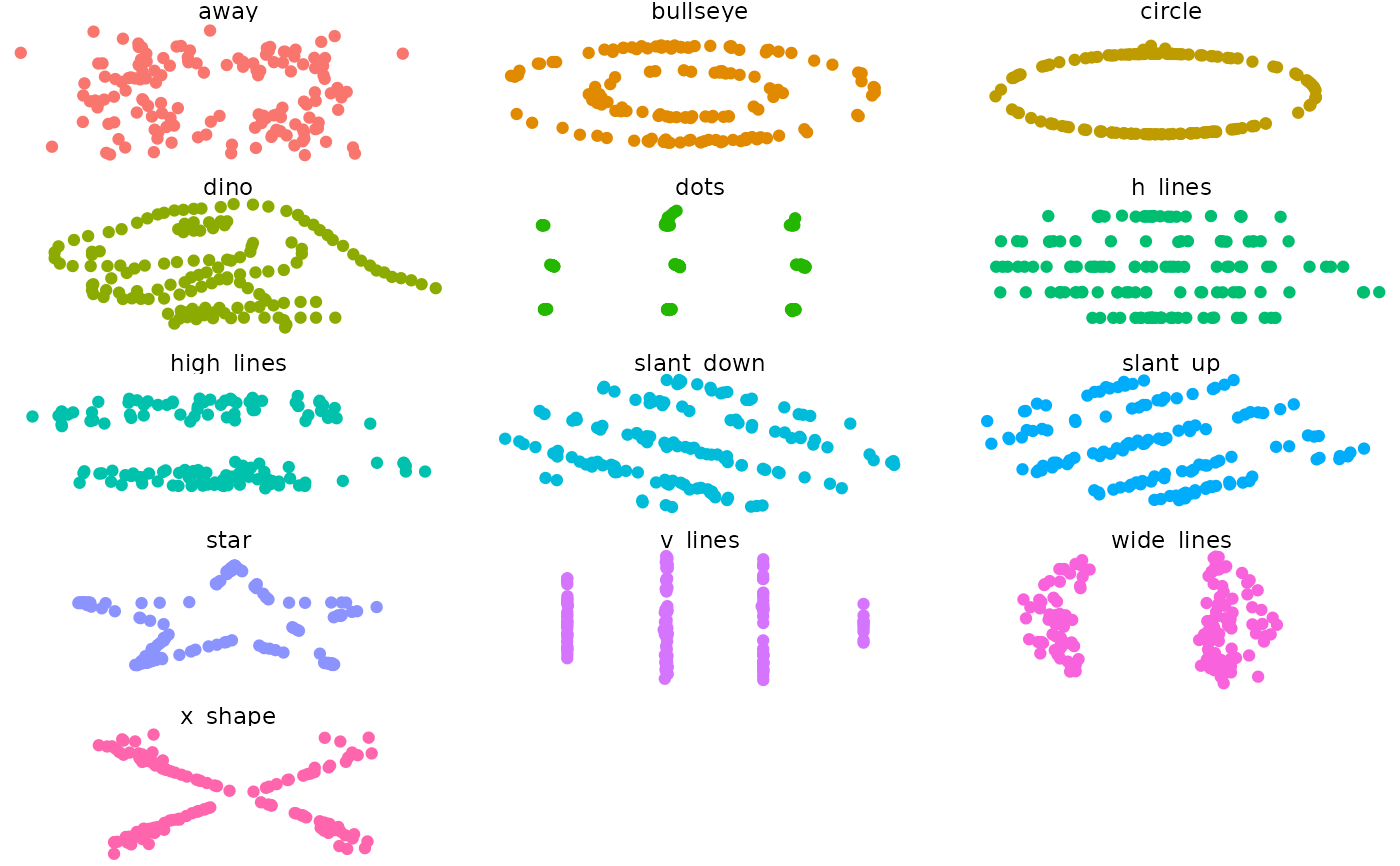A dataset demonstrating the utility of visualization. These 12 datasets are equal in standard measures: mean, standard deviation, and Pearson's correlation.

datasaurus_dozen

## Format

A data frame with 1846 rows and 3 variables:

• dataset: indicates which dataset the data are from

• x: x-values

• y: y-values

Matejka, J., & Fitzmaurice, G. (2017). Same Stats, Different Graphs: Generating Datasets with Varied Appearance and Identical Statistics through Simulated Annealing. CHI 2017 Conference proceedings: ACM SIGCHI Conference on Human Factors in Computing Systems. Retrieved from https://www.autodeskresearch.com/publications/samestats.

## Examples

if(require(ggplot2)){
ggplot(datasaurus_dozen, aes(x=x, y=y, colour=dataset))+
geom_point()+
theme_void()+
theme(legend.position = "none")+
facet_wrap(~dataset, ncol=3)
}